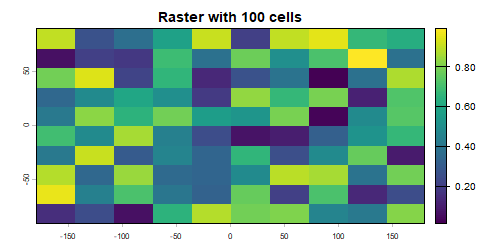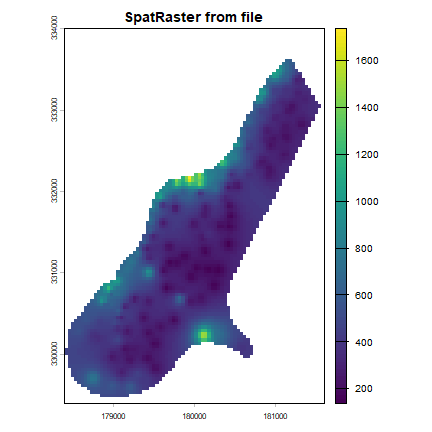# Creating SpatRaster objects¶

A `SpatRaster` can easily be created from scratch using the function `rast`. The default settings will create a global raster data structure with a longitude/latitude coordinate reference system and 1 by 1 degree cells. You can change these settings by providing additional arguments such as xmin, nrow, ncol, and/or crs, to the function. You can also change these parameters after creating the object. If you set the projection, this is only to properly define it, not to change it. To transform a `SpatRaster` to another coordinate reference system (projection) you can use the function `warp`.

Here is an example of creating and changing a `SpatRaster` object ‘r’ from scratch.

SpatRaster with default geometry parameters

```library(terra)
## terra 1.7.62
x <- rast()
x
## class       : SpatRaster
## dimensions  : 180, 360, 1  (nrow, ncol, nlyr)
## resolution  : 1, 1  (x, y)
## extent      : -180, 180, -90, 90  (xmin, xmax, ymin, ymax)
## coord. ref. : lon/lat WGS 84 (CRS84) (OGC:CRS84)
```

With other parameters

```x <- rast(ncol=36, nrow=18, xmin=-1000, xmax=1000, ymin=-100, ymax=900)
res(x)
##  55.55556 55.55556
```

Change the spatial resolution of an existing object

```res(x) <- 100
res(x)
##  100 100
ncol(x)
##  20
# change the numer of columns (affects resolution)
ncol(x) <- 18
ncol(x)
##  18
res(x)
##  111.1111 100.0000
```

Set the coordinate reference system (CRS) (define the projection)

```crs(x) <- "+proj=utm +zone=48 +datum=WGS84"
x
## class       : SpatRaster
## dimensions  : 10, 18, 1  (nrow, ncol, nlyr)
## resolution  : 111.1111, 100  (x, y)
## extent      : -1000, 1000, -100, 900  (xmin, xmax, ymin, ymax)
## coord. ref. : +proj=utm +zone=48 +datum=WGS84 +units=m +no_defs
```

The object `x` created in the example above only consist of a “skeleton”, that is, we have defined the number of rows and columns, and where the raster is located in geographic space, but there are no cell-values associated with it. Setting and accessing values is illustrated below.

```r <- rast(ncol=10, nrow=10)
ncell(r)
##  100
hasValues(r)
##  FALSE
# use the 'values' function, e.g.,
values(r) <- 1:ncell(r)
# or
set.seed(0)
values(r) <- runif(ncell(r))
hasValues(r)
##  TRUE
sources(r)
##  ""
values(r)[1:10]
##   0.8966972 0.2655087 0.3721239 0.5728534 0.9082078 0.2016819 0.8983897
##   0.9446753 0.6607978 0.6291140
plot(r, main='Raster with 100 cells')
```In some cases, for example when you change the number of columns or rows, you will lose the values associated with the `SpatRaster` if there were any (or the link to a file if there was one). The same applies, in most cases, if you change the resolution directly (as this can affect the number of rows or columns). Values are not lost when changing the extent as this change adjusts the resolution, but does not change the number of rows or columns.

```hasValues(r)
##  TRUE
res(r)
##  36 18
dim(r)
##  10 10  1
xmax(r)
##  180
# change the maximum x coordinate of the extent (bounding box) of the SpatRaster
xmax(r) <- 0
hasValues(r)
##  TRUE
res(r)
##  18 18
dim(r)
##  10 10  1
ncol(r) <- 6
hasValues(r)
##  FALSE
res(r)
##  30 18
dim(r)
##  10  6  1
xmax(r)
##  0
```

The function `terra` also allows you to create a `SpatRaster` from another object, including another `SpatRaster`, or `SpatRaster` objects from the “terra” package.

It is more common, however, to create a `SpatRaster` object from a file. The raster package can use raster files in several formats, including some ‘natively’ supported formats and other formats via the `rgdal` package. Supported formats for reading include GeoTIFF, ESRI, ENVI, and ERDAS. Most formats supported for reading can also be written to.

```# get the name of an example file installed with the package
# do not use this construction of your own files
filename <- system.file("ex/meuse.tif", package="terra")
filename
##  "C:/soft/R/R-4.3.2/library/terra/ex/meuse.tif"
r <- rast(filename)
sources(r)
##  "C:/soft/R/R-4.3.2/library/terra/ex/meuse.tif"
hasValues(r)
##  TRUE
plot(r, main='SpatRaster from file')
```Multi-layer objects can be created in memory (from `SpatRaster` objects) or from files.

```# create three identical SpatRaster objects
r1 <- r2 <- r3 <- rast(nrow=10, ncol=10)
# Assign random cell values
values(r1) <- runif(ncell(r1))
values(r2) <- runif(ncell(r2))
values(r3) <- runif(ncell(r3))
```

Combine the three SpatRaster objects into a single object with three layers.

```s <- c(r1, r2, r3)
s
## class       : SpatRaster
## dimensions  : 10, 10, 3  (nrow, ncol, nlyr)
## resolution  : 36, 18  (x, y)
## extent      : -180, 180, -90, 90  (xmin, xmax, ymin, ymax)
## coord. ref. : lon/lat WGS 84 (CRS84) (OGC:CRS84)
## source(s)   : memory
## names       :      lyr.1,      lyr.1,      lyr.1
## min values  : 0.01307758, 0.02778712, 0.06380247
## max values  : 0.99268406, 0.98156346, 0.99607737
nlyr(s)
##  3
```

Create a multilayer SpatRaster from file

```filename <- system.file("ex/logo.tif", package="terra")
filename
##  "C:/soft/R/R-4.3.2/library/terra/ex/logo.tif"
b <- rast(filename)
b
## class       : SpatRaster
## dimensions  : 77, 101, 3  (nrow, ncol, nlyr)
## resolution  : 1, 1  (x, y)
## extent      : 0, 101, 0, 77  (xmin, xmax, ymin, ymax)
## coord. ref. : Cartesian (Meter)
## source      : logo.tif
## colors RGB  : 1, 2, 3
## names       : red, green, blue
## min values  :   0,     0,    0
## max values  : 255,   255,  255
nlyr(b)
##  3
```

Extract a layer

```r <- b[]
```